﻿ 财政支出结构对居民消费的动态影响———基于DSGE的实证分析文章快速检索 高级检索

Dynamic effect of government spending structure on household consumption——Empirical analysis based on DSGE model
School of International Trade and Economics, University of International Business and Economics, Beijing 100029, China
Abstract:We divide fiscal spending into productive spending and consumer spending which are incorporated into the production function and consumption function, respectively, then establish dynamic stochastic general equilibrium (DSGE) model by considering and without considering the effect of household consumption habit, respectively. The analysis results for solving the models show that the total effect of fiscal spending on household consumption depends on the relative size of two types of fiscal spendings. If the shock of productive spending is greater than that of consumer spending, then the fiscal spending crowds in the household consumption. Otherwise, the fiscal spending crowds out the household consumption. Especially, when we consider the consumption habit, the responses of consumption, output, employment and investment to the fiscal spending are hump-shaped. This is consistent with the empirical facts. Finally, we make some policy recommendations for the fiscal spending structure optimization and adjustment.
Key words: fiscal spending     household consumption     consumption habit     dynamic stochastic general equilibrium (DSGE)

0 引言

1 模型的构建

1)代表性家庭

 $\begin{equation} TC_{t}=C_{t}G_{ct}^{\rho} \end{equation}$ (1)
$C_{t}$为居民消费,$G_{ct}$为政府消费性支出, $\rho$表示居民消费与政府支出的关系系数, 实证表明这种非线性居民消费函数在中国比较适用. $0<\beta<1$为家庭效用的期望折现因子; $0<h<1$ 为消费习惯形成参数, 测度了代表性家庭的消费惯性; $\chi$为消费和劳动的相对权值,$\eta$ 为Frisch劳动供给弹性的倒数,$\gamma$ 是居民跨期消费替代弹性的倒数. $E_{0}$ 表示基于第0 期信息的数学期望.

 $\begin{equation} C_{t}+I_{t}+G_{t}=Y_{t} \end{equation}$ (2)

2)厂商

 $\begin{equation} Y_{t}=A_{t}K_{t-1}^{\alpha}N_{t}^{1-\alpha}G_{gt}^{\theta} \end{equation}$ (3)

 $\begin{equation} \ln A_{t+1}=(1-\phi_{A})\ln\overline{A}+\phi_{A}\ln A_{t}+\varepsilon_{At} \end{equation}$ (4)

 $\begin{equation} K_{t+1}=(1-\delta)K_{t}+I_{t} \end{equation}$ (5)

3)政府部门 假设政府的支出全部来源于税收,不考虑转移支付,并且是以总量税的形式获取,则政府部门的均衡关系如下: $G_{t}=τY_{t}$. 其中,$\tau$代表总量税税率,外生给定. 因此,政府支出冲击会通过影响居民的效用函数,对居民行为的选择造成影响,进而影响到消费、投资和产出等经济变量,甚至整个经济的波动.同时将政府支出分为消费性支出和生产性支出,满足$G_{t}=G_{ct}+G_{gt}$,且
 $\begin{equation}G_{ct}=\mu G_{t}\end{equation}$ (6)
$G_{gt}=(1-\mu)G_{t}$,其中 $\mu$为消费性支出占政府总支出的比例.

 $\begin{equation}\ln G_{g,t+1}=(1-\phi_{G}) ln\overline{G_{g}}+\phi_{G} lnG_{gt}+\varepsilon_{ggt}\end{equation}$ (7)
 $\begin{equation}\ln G_{c,t+1}=(1-\phi_{G}) ln\overline{G_{c}}+\phi_{G} lnG_{ct}+\varepsilon_{gct}\end{equation}$ (8)

s.t. $$TC_{t}=C_{t}G_{ct}^{\rho},$$$$C_{t}+K_{t+1}+(1-\delta)K_{t}+G_{t}=A_{t}K_{t-1}^{\alpha}N_{t}^{1-\alpha}G_{gt}^{\theta},$$$$\ln A_{t+1}=(1-\phi_{A})\ln\overline{A}+\phi_{A}\ln A_{t}+\varepsilon_{At},$$$$\ln G_{g,t+1}=(1-\phi_{G})\ln\overline{G_{g}}+\phi_{G}\ln G_{gt}+\varepsilon_{ggt},$$$$\ln G_{c,t+1}=(1-\phi_{G})\ln\overline{G_{c}}+\phi_{G}\ln G_{ct}+\varepsilon_{gct}.$$

 $\begin{equation}(TC_{t}-hTC_{t-1})^{-\gamma}G_{ct}^{\rho}-\beta hE_{t}[(TC_{t+1}-hTC_{t})^{-\gamma}G_{ct}^{\rho}]=\lambda_{t}\end{equation}$ (9)
 $\begin{equation}\chi N_{t}^{\eta}=(1-\alpha)\lambda_{t}\frac{Y_{t}}{N_{t}}\end{equation}$ (10)
 $\begin{equation}\beta E_{t}\bigg[\lambda_{t+1}\bigg(1-\delta+\alpha\frac{Y_{t+1}}{K_{t}}\bigg)\bigg]=\lambda_{t}\end{equation}$ (11)
$\lambda_{t}>0$为对应约束条件的拉格朗日乘子,测度了居民消费的边际效用. 其中(11)式的稳态形式为βR=1,其中$R=1-\delta+\alpha \frac{Y}{K}$ 为资本收益率.

4)竞争性均衡

1)厂商生产参数($\alpha$、$\theta$、$\mu$)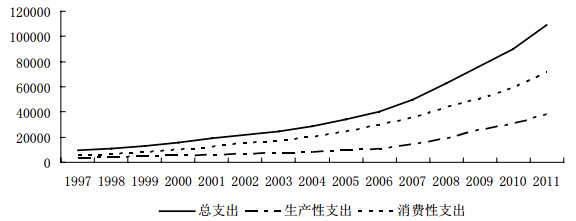图 1 政府支出类型变动趋势

2)家庭偏好参数($\beta$、$h$、$\eta$、$\gamma$、$\chi$、$\rho$)

3)有关技术冲击、政府冲击的参数($\phi_{A}$、$\sigma_{A\varepsilon}$、$\phi_{G}$、$\sigma_{gg\varepsilon}$、$\sigma_{gc\varepsilon}$) 首先,由生产函数估计,计算出"索洛剩余",见下式: $$\ln A_{t}=\ln(Y_{t}/N_{t})-0.36\ln(K_{t}/N_{t})-0.18\ln G_{gt},$$ 可以得到残差序列$A_{t}$,由于$A_{t}$满足一阶自回归过程,经过回归得到技术冲击一阶自回归的系数$\phi_{A}= 0.825$、及标准差$\sigma_{A\varepsilon} =1.98%$.

4) 其他参数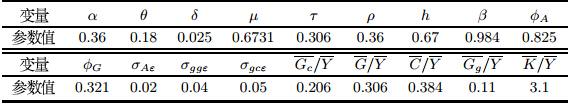2.2 贝叶斯估计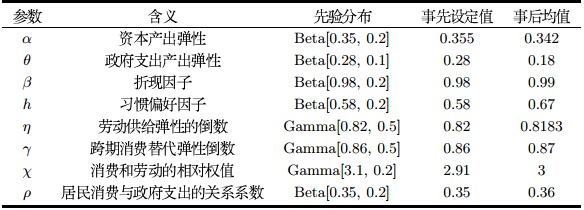3 两类财政支出冲击的动态效应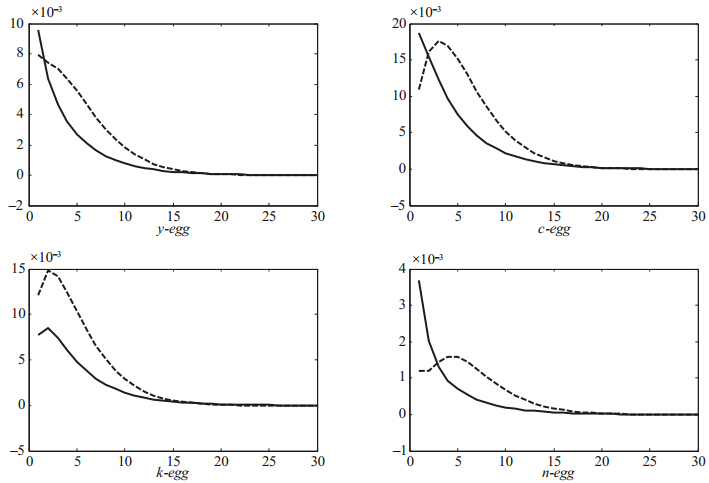注: 实线和虚线分别表示$h=0$和$h=0.67$时的情形.$y,c,k,n$分别表示总产出、消费、资本和就业.图 2 经济变量对财政生产性支出冲击的脉冲响应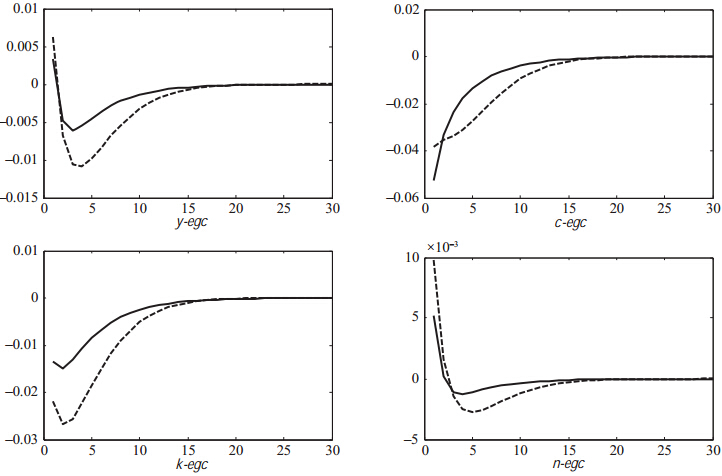注: 实线和虚线分别表示$h=0$和$h=0.67$时的情形.$y,c,k,n$分别表示总产出、消费、资本和就业.图 3 经济变量对财政消费性支出冲击的脉冲响应
3.1 财政生产性支出冲击对经济变量的动态效应

3.2 财政消费性支出冲击对经济变量的动态效应

4 结论

  Davig T, Leeper E M. Expectations and fiscal stimulus[R]. CAEPR Working Paper No. 006-2009. http://ssrn.com /abstract=1416885, http://dx.doi.org/10.2139/ssrn.1416885.  Mountford A, Uhlig H. What are the effects of fiscal policy shocks?[J]. Journal of Applied Econometrics, 2009, 24(6): 960-992.  Gali J, López-Salido J D, Vallés J. Understanding the effects of government spending on consumption[J]. Journal of the European Economic Association, 2007, 5(1): 227-270.  Blanchard O, Perotti R. An empirical characterization of the dynamic effects of changes in government spending and taxes on output[J]. The Quarterly Journal of Economics, 2002, 117(4): 1329-1368.  Wolff G B, Tenhofen J, Heppke-Falk K H. The macroeconomic effects of exogenous fiscal policy shocks in Germany: A disaggregated SVAR analysis[R]. Discussion Paper Series 1, 2006.  李广众.政府支出与居民消费:替代还是互补[J].世界经济, 2005, 5(2): 38-45.Li Guangzhong. Government spending and private consumption: Alternative or complement[J]. World Economy, 2005, 5(2): 38-45.  Coenen G, Straub R. Does government spending crowd in private consumption? Theory and empirical evidence for the Euro Area[J]. International Finance, 2005, 8(3): 435-470.  黄赜琳.中国经济周期特征和财政政策效应——-一个基于三部门RBC模型的实证分析[J].经济研究, 2005, 6(3): 27-39.Huang Zelin. Economic cycle characteristics and the effect of fiscal policy in China —— An empirical analysis based on the three sector model of RBC[J]. Economic Research, 2005, 6(3): 27-39.  Leeper E M, Plante M, Traum N. Dynamics of fiscal financing in the United States[J]. Journal of Econometrics, 2010, 156(2): 304-321.  Linnemann L. The effect of government spending on private consumption: A puzzle?[J]. Journal of Money, Credit and Banking, 2006, 38(7): 1715-1735.  贾俊雪,郭庆旺.财政支出类型、财政政策作用机理与最优财政货币规则[J].世界经济, 2012, 11(1): 3-30.Jia Junxue, Guo Qingwang. Types of fiscal expenditure, fiscal policy action mechanism and optimal monetary and fiscal rules[J]. World Economy, 2012, 11(1): 3-30.  单豪杰,师博.中国工业部门的资本回报率: 1978-2006[J].产业经济研究, 2008, 6(3): 26-37.Shan Haojie, Shi Bo. Capital return rate of Chinese industry: 1978-2006[J]. Industry Economy Research, 2008, 6(3): 26-37.  杜清源,龚六堂.带"金融加速器"的RBC 模型[J].金融研究, 2005, 4(2): 16-30.Du Qingyuan, Gong Liutang. RBC model with financial accelerator[J]. Financial Research, 2005, 4(2): 16-30.  Gilchrist S, Saito M. Expectations, asset prices, and monetary policy: The role of learning[R]. National Bureau of Economic Research, 2006.  李宾,曾志雄.中国全要素生产率变动的再测算: 1978-2007[J].数量经济技术经济研究, 2009, 3(1): 3-15.Li Bin, Ceng Zhixiong. Calculation of TFP change in China: 1978-2007[J]. Quantitative and Technical Economics, 2009, 3(1): 3-15.  Davig T, Leeper E M. Monetary-fiscal policy interactions and fiscal stimulus[J]. European Economic Review, 2011, 55(2): 211-227.  Baxter M, King R. Fiscal policy in general equilibrium[J]. American Economic Review, 1993, 8(3): 315-334.  Fatás A, Mihov I. The effects of fiscal policy on consumption and employment: Theory and evidence[R]. INSEAD, 2001.  Monacelli T, Perotti R. Fiscal policy, wealth effects, and markups[R]. National Bureau of Economic Research, 2008.
0

#### 文章信息

WANG Yu-feng, LIU Shu-lin

Dynamic effect of government spending structure on household consumption——Empirical analysis based on DSGE model

Systems Engineering - Theory & practice, 2015, 35(2): 300-307.